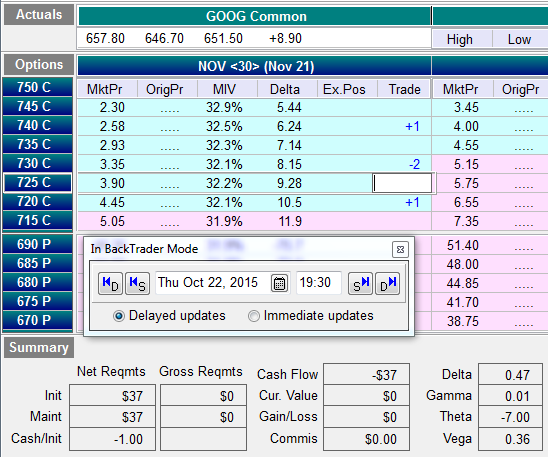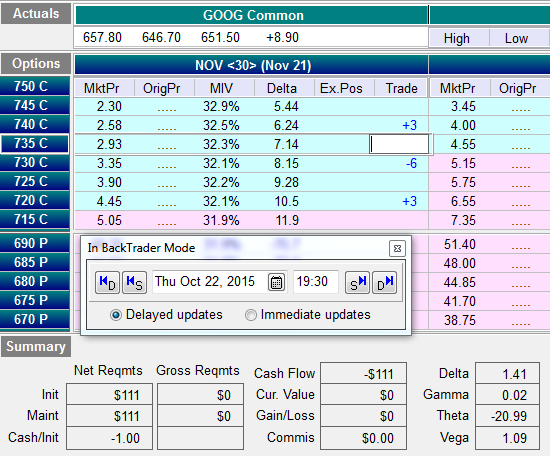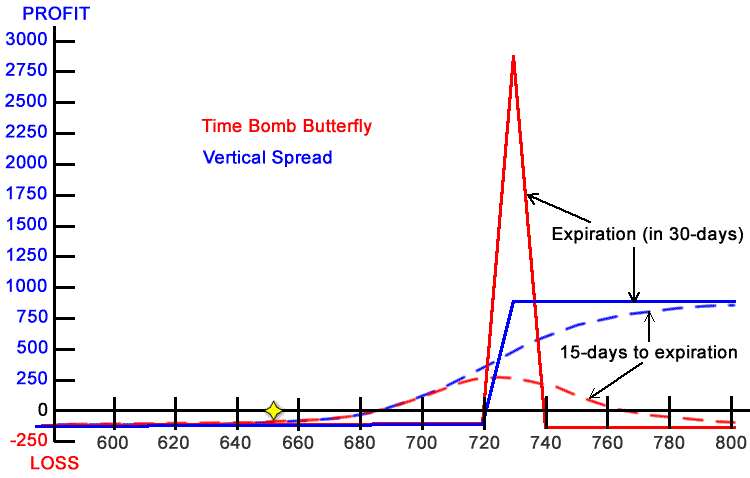Mark Fenton wrote an article about the Time Bomb Butterfly. Mark said “one of the best butterfly strategies is the time bomb butterfly.” There isn't one best strategy as each strategy has its pros and cons. The time bomb butterfly suffers from a very low probability of profit and negative expected return. I wondered what other strategy could you use instead of a time bomb butterfly that would have similar performance?

Vertical spreads are very flexible strategies. You can place them in-the-money and have a higher probability of making money with lower returns or you can place them out-of-the-money with a lower probability and higher return. Let's see how an out-of-the-money vertical spread compares to the time bomb butterfly.

Mark went back to Oct 22, 2015 and bought a 720/730/740 GOOG butterfly. My prices in OptionVue were \$0.37 per butterfly. Here's what it looks like in the OptionVue Matrix:I decided to use similar strikes for the vertical spread, using the 720/730 Call Debit spread for \$112:One problem I see is the vertical spread is three times the cost. It's still not expensive, but let's triple the size of the time bomb butterfly to compare apples to apples.Now we can compare the two trades as they are nearly the same debit. The vertical spread has twice the positive delta, three times the negative theta and six times the positive vega. What does that all mean? Let's look at the risk chart of the two trades to get an idea:Notice that in 15-days (T+15) both trades have nearly the exact same profit up until GOOG reaches \$710. As GOOG price goes above \$710, the Vertical Spread keeps rising while the butterfly is losing profits.

## Probability of Profit and Expected Return

In 15-days, the time bomb butterfly has a probability of profit of 27% and an expected return of -\$8. The Vertical Spread has a probability of profit of 27% and an expected return of +\$23.

At expiration, the time bomb butterfly has a probability of profit of 5% and an expected return of -\$9. The Vertical Spread has a probability of profit of 15% and an expected return of +\$22.